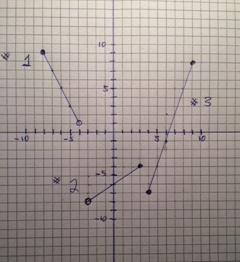### Writing Equations for Piecewise Functions

Writing equations for piecewise functions:First take a given line and find the slope, then use the point slope formula to write an equation. Then define the domain over which the function occurs.

Lets do this for example #1:

the slope of the line is down 2, over 1 which is -2. The top point is (-8,9) using the point slope equation Y-y1=M(X-x1) we have Y-9=-2(X- -8) rearranging add 9 to both sides and we get Y=-2(X+8)+9 The domain values or X values over which this function ocurrs are   -8<_X<-4

Example #2:

Using point (-3,-8)  The line is a positive slope of up 2 over 3 or 2/3

using point slope equation: Y- -8=2/3(X- -3) This rearranges into

Y+8=2/3(X+3)  then add -8 to both sides to get Y=2/3(X+3)-8 the domain is -3<X<_3

Example #3:

Use either point. I’ll use(9,8) the line has a slope of up 3 over 2 or 3/2  Using the point slope formula:

Y-8=3/2(X-9)   now add 8 to both sides

Y=3/2(X-9) + 8  The domain is 4<_X<_9